The REG Procedure
 MTEST Statement
<label:> MTEST <equation <, ..., equation>> < / options> ;

where each equation is a linear function composed of coefficients and variable names. The label is optional.

The MTEST statement is used to test hypotheses in multivariate regression models where there are several dependent variables fit to the same regressors. If no equations or options are specified, the MTEST statement tests the hypothesis that all estimated parameters except the intercept are zero.

The hypotheses that can be tested with the MTEST statement are of the form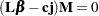whereis a linear function on the regressor side,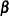is a matrix of parameters,is a column vector of constants,is a row vector of ones, and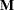is a linear function on the dependent side. The special case where the constants are zero is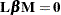See the section Multivariate Tests for more details.

Each linear function extends across either the regressor variables or the dependent variables. If the equation is across the dependent variables, then the constant term, if specified, must be zero. The equations for the regressor variables form thematrix andvector in the preceding formula; the equations for dependent variables form thematrix. If no equations for the dependent variables are given, PROC REG uses an identity matrix for, testing the same hypothesis across all dependent variables. If no equations for the regressor variables are given, PROC REG forms a linear function corresponding to a test that all the nonintercept parameters are zero.

As an example, consider the following statements:

```model y1 y2 y3=x1 x2 x3;
mtest x1,x2;
mtest y1-y2, y2 -y3, x1;
mtest y1-y2;
```

The first MTEST statement tests the hypothesis that the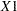and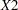parameters are zero for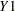,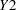, and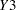. In addition, the second MTEST statement tests the hypothesis that theparameter is the same for all three dependent variables. For the same model, the third MTEST statement tests the hypothesis that all parameters except the intercept are the same for dependent variablesand.

You can specify the following options in the MTEST statement:

CANPRINT

displays the canonical correlations for the hypothesis combinations and the dependent variable combinations. If you specify

```mtest / canprint;
```

the canonical correlations between the regressors and the dependent variables are displayed.

DETAILS

displays thematrix and various intermediate calculations.

MSTAT=FAPPROX
MSTAT=EXACT

specifies the method of evaluating the multivariate test statistics. The default is MSTAT=FAPPROX, which specifies that the multivariate tests are evaluated by using the usual approximations based on thedistribution, as discussed in the "Multivariate Tests" section in Chapter 4, Introduction to Regression Procedures. Alternatively, you can specify MSTAT=EXACT to compute exact p-values for three of the four tests (Wilks’ lambda, the Hotelling-Lawley trace, and Roy’s greatest root) and an improvedapproximation for the fourth (Pillai’s trace). While MSTAT=EXACT provides better control of the significance probability for the tests, especially for Roy’s greatest root, computations for the exact p-values can be appreciably more demanding, and are in fact infeasible for large problems (many dependent variables). Thus, although MSTAT=EXACT is more accurate for most data, it is not the default method.

PRINT

displays the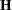and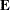matrices.Previous Page | Next Page | Top of Page